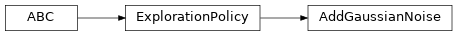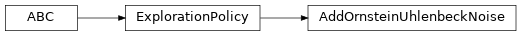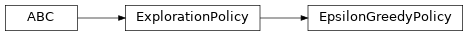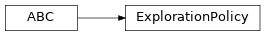# garage.np.exploration_policies¶

Exploration strategies which use NumPy as a numerical backend.

class AddGaussianNoise(env_spec, policy, total_timesteps, max_sigma=1.0, min_sigma=0.1, decay_ratio=1.0)[source]Add Gaussian noise to the action taken by the deterministic policy.

Parameters
• env_spec (EnvSpec) – Environment spec to explore.

• policy (garage.Policy) – Policy to wrap.

• total_timesteps (int) – Total steps in the training, equivalent to max_episode_length * n_epochs.

• max_sigma (float) – Action noise standard deviation at the start of exploration.

• min_sigma (float) – Action noise standard deviation at the end of the decay period.

• decay_ratio (float) – Fraction of total steps for epsilon decay.

get_action(self, observation)[source]

Get action from this policy for the input observation.

Parameters

observation (numpy.ndarray) – Observation from the environment.

Returns

Actions with noise. List[dict]: Arbitrary policy state information (agent_info).

Return type

np.ndarray

get_actions(self, observations)[source]

Get actions from this policy for the input observation.

Parameters

observations (list) – Observations from the environment.

Returns

Actions with noise. List[dict]: Arbitrary policy state information (agent_info).

Return type

np.ndarray

update(self, episode_batch)[source]

Update the exploration policy using a batch of trajectories.

Parameters

episode_batch (EpisodeBatch) – A batch of trajectories which were sampled with this policy active.

get_param_values(self)[source]

Get parameter values.

Returns

Values of each parameter.

Return type

list or dict

set_param_values(self, params)[source]

Set param values.

Parameters

params (np.ndarray) – A numpy array of parameter values.

reset(self, dones=None)

Reset the state of the exploration.

Parameters

dones (List[bool] or numpy.ndarray or None) – Which vectorization states to reset.

class AddOrnsteinUhlenbeckNoise(env_spec, policy, *, mu=0, sigma=0.3, theta=0.15, dt=0.01, x0=None)[source]An exploration strategy based on the Ornstein-Uhlenbeck process.

The process is governed by the following stochastic differential equation.

$dx_t = -\theta(\mu - x_t)dt + \sigma \sqrt{dt} \mathcal{N}(\mathbb{0}, \mathbb{1}) # noqa: E501$
Parameters
• env_spec (EnvSpec) – Environment to explore.

• policy (garage.Policy) – Policy to wrap.

• mu (float) – $$\mu$$ parameter of this OU process. This is the drift component.

• sigma (float) – $$\sigma > 0$$ parameter of this OU process. This is the coefficient for the Wiener process component. Must be greater than zero.

• theta (float) – $$\theta > 0$$ parameter of this OU process. Must be greater than zero.

• dt (float) – Time-step quantum $$dt > 0$$ of this OU process. Must be greater than zero.

• x0 (float) – Initial state $$x_0$$ of this OU process.

reset(self, dones=None)[source]

Reset the state of the exploration.

Parameters

dones (List[bool] or numpy.ndarray or None) – Which vectorization states to reset.

get_action(self, observation)[source]

Return an action with noise.

Parameters

observation (np.ndarray) – Observation from the environment.

Returns

An action with noise. dict: Arbitrary policy state information (agent_info).

Return type

np.ndarray

get_actions(self, observations)[source]

Return actions with noise.

Parameters

observations (np.ndarray) – Observation from the environment.

Returns

Actions with noise. List[dict]: Arbitrary policy state information (agent_info).

Return type

np.ndarray

update(self, episode_batch)

Update the exploration policy using a batch of trajectories.

Parameters

episode_batch (EpisodeBatch) – A batch of trajectories which were sampled with this policy active.

get_param_values(self)

Get parameter values.

Returns

Values of each parameter.

Return type

list or dict

set_param_values(self, params)

Set param values.

Parameters

params (np.ndarray) – A numpy array of parameter values.

class EpsilonGreedyPolicy(env_spec, policy, *, total_timesteps, max_epsilon=1.0, min_epsilon=0.02, decay_ratio=0.1)[source]ϵ-greedy exploration strategy.

Select action based on the value of ϵ. ϵ will decrease from max_epsilon to min_epsilon within decay_ratio * total_timesteps.

At state s, with probability 1 − ϵ: select action = argmax Q(s, a) ϵ : select a random action from an uniform distribution.

Parameters
• env_spec (garage.envs.env_spec.EnvSpec) – Environment specification.

• policy (garage.Policy) – Policy to wrap.

• total_timesteps (int) – Total steps in the training, equivalent to max_episode_length * n_epochs.

• max_epsilon (float) – The maximum(starting) value of epsilon.

• min_epsilon (float) – The minimum(terminal) value of epsilon.

• decay_ratio (float) – Fraction of total steps for epsilon decay.

get_action(self, observation)[source]

Get action from this policy for the input observation.

Parameters

observation (numpy.ndarray) – Observation from the environment.

Returns

An action with noise. dict: Arbitrary policy state information (agent_info).

Return type

np.ndarray

get_actions(self, observations)[source]

Get actions from this policy for the input observations.

Parameters

observations (numpy.ndarray) – Observation from the environment.

Returns

Actions with noise. List[dict]: Arbitrary policy state information (agent_info).

Return type

np.ndarray

update(self, episode_batch)[source]

Update the exploration policy using a batch of trajectories.

Parameters

episode_batch (EpisodeBatch) – A batch of trajectories which were sampled with this policy active.

get_param_values(self)[source]

Get parameter values.

Returns

Values of each parameter.

Return type

list or dict

set_param_values(self, params)[source]

Set param values.

Parameters

params (np.ndarray) – A numpy array of parameter values.

reset(self, dones=None)

Reset the state of the exploration.

Parameters

dones (List[bool] or numpy.ndarray or None) – Which vectorization states to reset.

class ExplorationPolicy(policy)[source]

Bases: abc.ABCPolicy that wraps another policy to add action noise.

Parameters

policy (garage.Policy) – Policy to wrap.

abstract get_action(self, observation)[source]

Return an action with noise.

Parameters

observation (np.ndarray) – Observation from the environment.

Returns

An action with noise. dict: Arbitrary policy state information (agent_info).

Return type

np.ndarray

abstract get_actions(self, observations)[source]

Return actions with noise.

Parameters

observations (np.ndarray) – Observation from the environment.

Returns

Actions with noise. List[dict]: Arbitrary policy state information (agent_info).

Return type

np.ndarray

reset(self, dones=None)[source]

Reset the state of the exploration.

Parameters

dones (List[bool] or numpy.ndarray or None) – Which vectorization states to reset.

update(self, episode_batch)[source]

Update the exploration policy using a batch of trajectories.

Parameters

episode_batch (EpisodeBatch) – A batch of trajectories which were sampled with this policy active.

get_param_values(self)[source]

Get parameter values.

Returns

Values of each parameter.

Return type

list or dict

set_param_values(self, params)[source]

Set param values.

Parameters

params (np.ndarray) – A numpy array of parameter values.